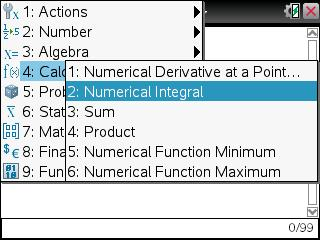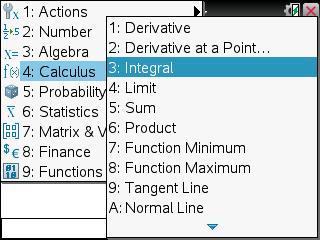Knowledge Base

Solution 25050: Calculating Integrals Using the TI-Nspire™ Family.

How do I calculate indefinite and definite (numerical) integrals using the TI-Nspire family?

To calculate definite (numerical) integrals using the TI-Nspire family, please follow the examples listed below.

Example: Calculate the definite (numerical) integral of x^3+x in the interval [1,3].

1) Press [home] [A] or choose to add a Calculator application.2) Press [menu]   on the TI-Nspire or [menu]   on the TI-Nspire CAS.3) Press  [tab]  [tab].
4) Press [X] [^]  [tab] [+] [X].
5) Press [tab] [X] [enter].Please Note: The TI-Nspire CAS family may also calculate indefinite integrals as it incorporates CAS (Computer Algebra System). To calculate indefinite integrals use the example below.

Example: Calculate the indefinite integral of x^3+x.